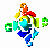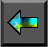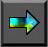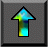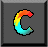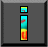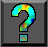Next: Chapter Notes Up: 4 Putting Components Together Previous: 4.7 Summary

# Exercises

1. Discuss ways in which modular design techniques are used in the design of an automobile engine, house, or suspension bridge.

2. Identify ways in which modularity ideas might apply to the management of an orchestra, educational institution, or company.

3.   Develop analytic models for the maximum throughput (in requests processed per second) supported by the centralized and distributed tuple space implementations outlined in Section 4.5.

4. Using a language that supports concurrent composition, such as CC++ \ or Fortran M, implement centralized and distributed tuple space modules. Study the performance of these modules in a simple parameter study problem, and relate performance to the models of Exercise 3.

5. Discuss ways in which the database search algorithm of Section 4.5 could be modified to avoid the central bottleneck inherent in a single manager.

6. An alternative parallel algorithm for the 2-D FFT considered in Section 4.4.1 assumes a fixed 2-D decomposition of data structures; hence, communication is required within each 1-D FFT. Use a performance model to contrast this algorithm with those described in the text. Assume that the communication costs for a 1-D FFT algorithm operating on N points on P processors are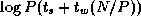.

7.   A problem comprises two components, A and B . A can be solved in 1000 seconds on computer C1 and in 5000 seconds on computer C2 ; B requires 4000 and 2000 seconds on C1 and C2 , respectively. The two computers are connected by a 1000-km optical fiber link that can transfer data at 100 MB/sec with a latency of 10 msec. The two components can execute concurrently but must transfer 10 MB of data 10,000 times during execution. Is it cheapest (in terms of computational resources consumed) to solve the problem on computer C1 , on computer C2 , or on both computers together?

8. A problem similar to that of Exercise 7 is found to use fewer computational resources when run on the two networked computers than on either computer alone. Your public relations office proposes to promote this as an example of superlinear speedup. Do you agree?

9. A climate model consists of an atmosphere and ocean model. At each step, the models both perform a finite difference computation and exchange five 2-D arrays. Develop performance models for two alternative parallel implementations, based on sequential and parallel composition respectively. Discuss the relative performance of the two implementations.

10. Determine the problem size and processor count regimes in which each of the three convolution algorithms described in Example 4.4 would be faster, assuming machine parameters characteristic of (a) a multicomputer and (b) an Ethernet-connected LAN.

11. The performance analysis of the pipelined algorithms considered in Section 4.4 did not take into account idle time incurred when starting up and shutting down pipelines. Refine the performance models to account for these costs. How many images must be processed before efficiency reaches 95 percent of that predicted in the absence of these costs?

12. Execute by hand for N=P=4 the matrix multiplication algorithm based on a 2-D decomposition described in Section 4.6.1.

13.   A simpler variant of the multiplication algorithm for matrices decomposed in two dimensions uses a ring rather than a tree for the broadcast operations. Use performance models to compare the two algorithm variants. (Note that a broadcast can be performed on a P -node bidirectional ring in approximately P/2 steps.)

14. Extend the analysis and comparison of Exercise 13 to account for competition for bandwidth in a 2-D mesh architecture.

15. Develop a performance model for the systolic matrix multiplication algorithm of Section 4.6, and use this to identify regimes in which this algorithm is faster than the simpler algorithm based on regular 2-D decompositions. Account for data reorganization costs.

16. Another matrix multiplication algorithm collects the matrices that are to be multiplied on a single processor. Use performance models to identify regimes in which this algorithm might be competitive. Conduct empirical studies to validate your analytic results.Next: Chapter Notes Up: 4 Putting Components Together Previous: 4.7 Summary